Courses

# Test: Mesh & Nodal Analysis

## 20 Questions MCQ Test Network Theory (Electric Circuits) | Test: Mesh & Nodal Analysis

Description
This mock test of Test: Mesh & Nodal Analysis for Electrical Engineering (EE) helps you for every Electrical Engineering (EE) entrance exam. This contains 20 Multiple Choice Questions for Electrical Engineering (EE) Test: Mesh & Nodal Analysis (mcq) to study with solutions a complete question bank. The solved questions answers in this Test: Mesh & Nodal Analysis quiz give you a good mix of easy questions and tough questions. Electrical Engineering (EE) students definitely take this Test: Mesh & Nodal Analysis exercise for a better result in the exam. You can find other Test: Mesh & Nodal Analysis extra questions, long questions & short questions for Electrical Engineering (EE) on EduRev as well by searching above.
QUESTION: 1

### Find the value of the currents I1, I2 and I3 flowing clockwise in the first, second and third mesh respectively.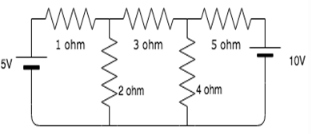Solution:

The three mesh equations are:
-3I1+2I2-5=0
2I1-9I2+4I3=0
4I2-9I3+10=0
Solving the equations, we get I1= 1.54A, I2=-0.189 and I3= -1.195A.

QUESTION: 2

### Find the value of the currents I1 and I2 flowing clockwise in the first and second mesh respectively.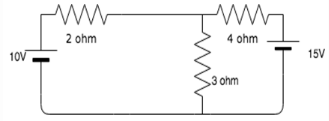Solution:

The two mesh equations are:
5I1-3I2=10
-3I1+7I2=-15
Solving the equations simultaneously, we get I1=0.96A and I2=-1.73A.

QUESTION: 3

### Find the value of V if the current in the 3 ohm resistor=0.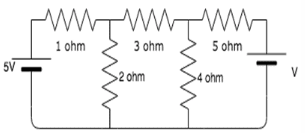Solution:

Taking the mesh currents in the three meshes as I1, I2 and I3, the mesh equations are:
3I1+0I2+0V=5
-2I1-4I2+0V=0
0I1+9I2+V=0
Solving these equations simultaneously and taking the value of I2=0, we get V=7.5V.

QUESTION: 4

Find the value of V1 if the current through the 1 ohm resistor=0A.​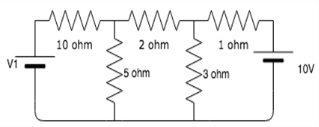Solution:

Taking I1, I2 and I3 as the currents in the three meshes and taking I3=0 since it is the current across the 1 ohm resistor, the three mesh equations are:
15I1-5I2=V1
-5I1+10I2+0V1=0
0I1-3I2+0V1=10
Solving these equations simultaneously we get V1= 83.33V.

QUESTION: 5

Calculate the mesh currents I1 and I2 flowing in the first and second meshes respectively.​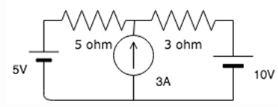Solution:

In this circuit, we have a super mesh present. Let I1 and I2 be the currents in loops in clockwise direction. The two mesh equations are:
I2-I1=3
-5I1-3I2=5
Solving these equations simultaneously, we get I1= -1.75A and I2= 1.25A.
Since no specific direction given so currents in loop 1 and loop 2 are 1.75A and 1.25A respectively.

QUESTION: 6

I1 is the current flowing in the first mesh. I2 is the current flowing in the second mesh and I3 is the current flowing in the top mesh. If all three currents are flowing in the clockwise direction, find the value of I1, I2 and I3.​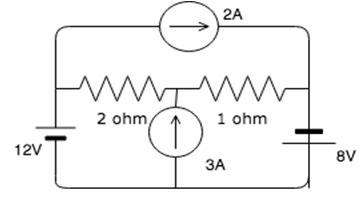Solution:

Explanation: The two meshes which contain the 3A current is a super mesh. The three mesh equations therefore are:
I3=2A
I2-I1=3
-2I1-I2=-26
Solving these equations simultaneously we get:
I1=7.67A, I2=10.67A and I3=2A.

QUESTION: 7

Calculate the mesh currents.​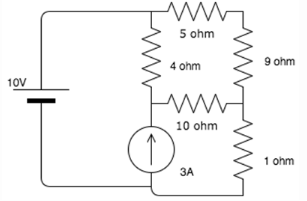Solution:

The two meshes which contain the 3A source, act as a supper mesh. The mesh equations are:
I1-I2=3
-11I1-4I2+14I3=-10
10I1+4I2-28I3=0
Solving these equations simultaneously, we get the three currents as 2A, 1A and 0.57A.

QUESTION: 8

Mesh analysis employs the method of ___________

Solution:

KVL employs mesh analysis to find the different mesh currents by finding the IR products in each mesh.

QUESTION: 9

Mesh analysis is generally used to determine _________

Solution:

Mesh analysis uses Kirchhoff’s Voltage Law to find all the mesh currents. Hence it is a method used to determine current.

QUESTION: 10

Mesh analysis can be used for __________

Solution:

If the circuit is not planar, the meshes are not clearly defined. In planar circuits, it is easy to draw the meshes hence the meshes are clearly defined.

QUESTION: 11

Find the value of the node voltage V. ​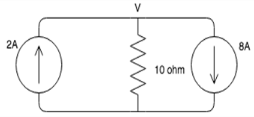Solution:

The node equation is:
-2+8+V/10=0 => 6 + v/10 = 0 => v = 10*6=>60v
Solving this equation, we get V=60V.

QUESTION: 12

Calculate the node voltages V1 and V2.​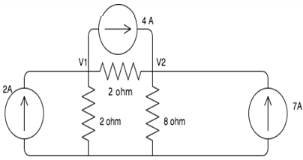Solution:

The nodal equations are:
2V1-V2=-4
-4V1+5V2=88
Solving these equations simultaneously, we get V1=11.33V and V2=26.67V.

QUESTION: 13

Find the node voltage V.​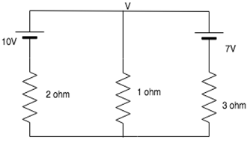Solution:

The nodal equation is:
(V-10)/2+(V-7)/3+V/1=0
Solving for V, we get V=4V.

QUESTION: 14

Calculate the node voltages.
​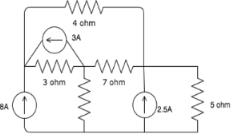Solution:

The nodal equations, considering V1, V2 and V3 as the first, second and third node respectively, are:
-8+(V1-V2)/3-3+(V1-V3)/4=0
3+V2+(V2-V3)/7+(V2-V1)/3=0
2.5+(V3-V2)/7+(V3-V1)/4+V3/5=0
Solving the equations simultaneously, we get V1=24.32V, V2=4.09V and V3=7.04V.

QUESTION: 15

Find the value of V1 and V2.​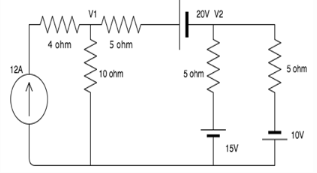Solution:

The nodal equations are:
0.3V1-0.2V2=16
-V1+3V2=-15
Solving these equations simultaneously, we get V1=64.28V and V2=16.42V.

QUESTION: 16

Nodal analysis is generally used to determine_______

Solution:

Nodal analysis uses Kirchhoff’s Current Law to find all the node voltages. Hence it is a method used to determine voltage.

QUESTION: 17

KCL is associated with_________

Solution:

KCL employs nodal analysis to find the different node voltages by finding the value if current in each branch.

QUESTION: 18

If there are 10 nodes in a circuit, how many equations do we get?

Solution:

The number of equations we get is always one less than the number of nodes in the circuit, hence for 10 nodes we get 9 equations.

QUESTION: 19

Nodal analysis can be applied for________

Solution:

Nodal analysis can be applied for both planar and non-planar networks since each node, whether it is planar or non-planar, can be assigned a voltage.

QUESTION: 20

How many nodes are taken as reference nodes in nodal analysis?

Solution:

In nodal analysis one node is treated as the reference node and the voltage at that point is taken as 0.